# Majority element in an array

Key takeaways

• A famous interview problem to learn problem-solving using various approaches and step-by-step time and space complexity optimization.
• The Boyer-Moore Voting algorithm is worth exploring, which uses fascinating insight from the problem to get the solution in place using a single loop and variables.

### Let’s understand the problem

You are given an array X[] consisting of n elements, write a program to find the majority element in an array i.e. return the number which appears more than n/2 times.

• Assume that the array is non-empty and the majority element always exists.
• A majority element is an element that appears more than n/2 times, so there is at most one such element.

Examples

Input: X[] = [2, 12, 2, 2, 2, 4, 2], Output: 2

Explanation: 2 is the majority element which appears 5 times.

Input: X[] = [4, 3, 4], Output: 4

Input: X[] = [1, 1, 1, 1, 1, 1], Output: 1

### Discussed solution approaches

• A brute force approach  using nested loops
• Using sorting
• Using the divide and conquer approach
• Using a hash table
• Using bit manipulation approach
• Using randomized algorithm
• Boyer-Moore voting algorithm

### A brute force approach  using nested loops

Solution Idea

A basic approach would be to check whether each element is the majority element or not. We can use two nested loops where the outer loop iterate over the array, and the inner loop counts the occurrences of each element. If we found an element with a frequency greater than n/2, that’s our majority element.

Solution Pseudocode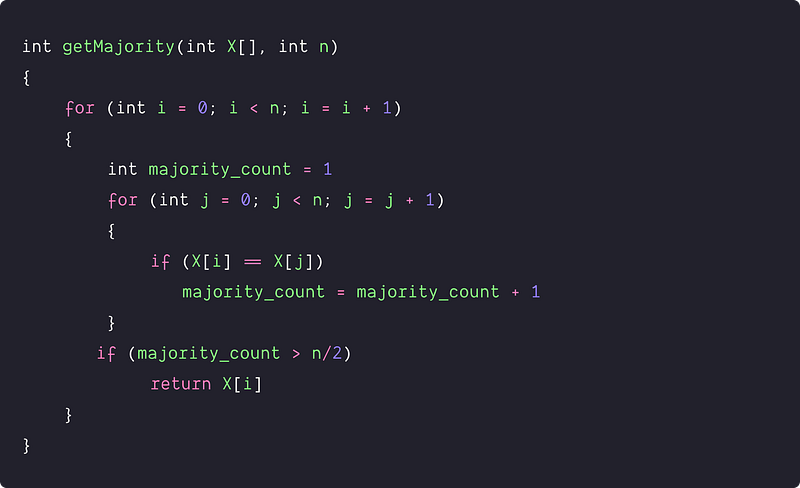Time and space complexity analysis

We are running two nested loops where each loop is running n times. Time Complexity = O(n²), Space Complexity = O(1). Can we improve the time complexity of the solution further? Let's think!

### Using sorting

Given in the problem statement, the majority element always exists in the array. So if we sort the array in increasing order, then the majority element will get grouped on adjacent indices. Can we access the majority element directly in the sorted array? (Think!)

If we divide the sorted array into two halves, each part contains n/2 elements each, and we also know that the majority element repeated more than n/2 times in the array. Therefore, if the majority element is present in the array, it must be present at the middle index of the sorted array. This is true for the cases when n is odd, and n is even.

Solution Pseudocode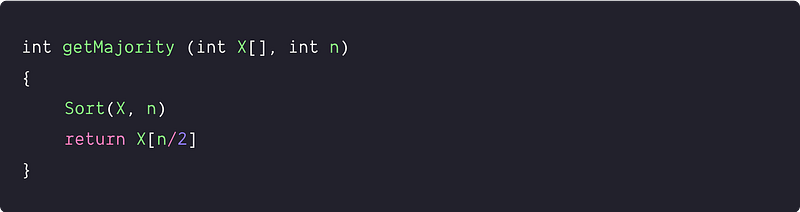Time and space complexity analysis

Suppose we are using an efficient O(nlogn) sorting algorithm heap sort. Time Complexity = Time complexity of heap sort + accessing the middle element = O(nlogn) + O(1) = O(nlogn)

Space complexity: O(1), heap sort the array in-place.

### Using the divide and conquer approach

Can we apply the divide and conquer approach? Here is an insight: If we divide the input array into two halves and recursively find the majority element of the left and right halves, then we can determine the global majority element in the linear time. (Think!)

Solution Steps

We define a helper function getMajority(int X[], int l, int r) which takes a left and right end of the current array as a parameter. The initial value of l = 0 and r = n-1.

Base case: This is the trivial case of the single element array when both the left and right ends are equal. We return this single element as the majority element i.e. if (l == r), return X[l].

Divide step: Divide the array into two equal halves by calculating the mid-value, i.e., mid = (r — l)/2 + l

Conquer step: we recursively calculate the majority element of the left and right halves and store them in the variable leftMajority and rightMajority.

• int leftMajority = getMajority (X, l, mid)
• int rightMajority = getMajority (X, mid + 1, r)

Combine step: If the majority elements of the left and right halves are equal, then it must be the global majority element and we return this value (Think!). Otherwise, one of them must be equal to the global majority element. To determine this, we count the frequency of the leftMajority and rightMajority and return the value with maximum frequency as the final output.

``````if (leftMajority == rightMajority)
return leftMajority

int leftCount = countFrequency(X,l, r, leftMajority)
int rightCount = countFrequency(X, l, r, rightMajority)

if(leftCount > rightCount)
return leftCount
else
return rightCount
``````

Solution Pseudocode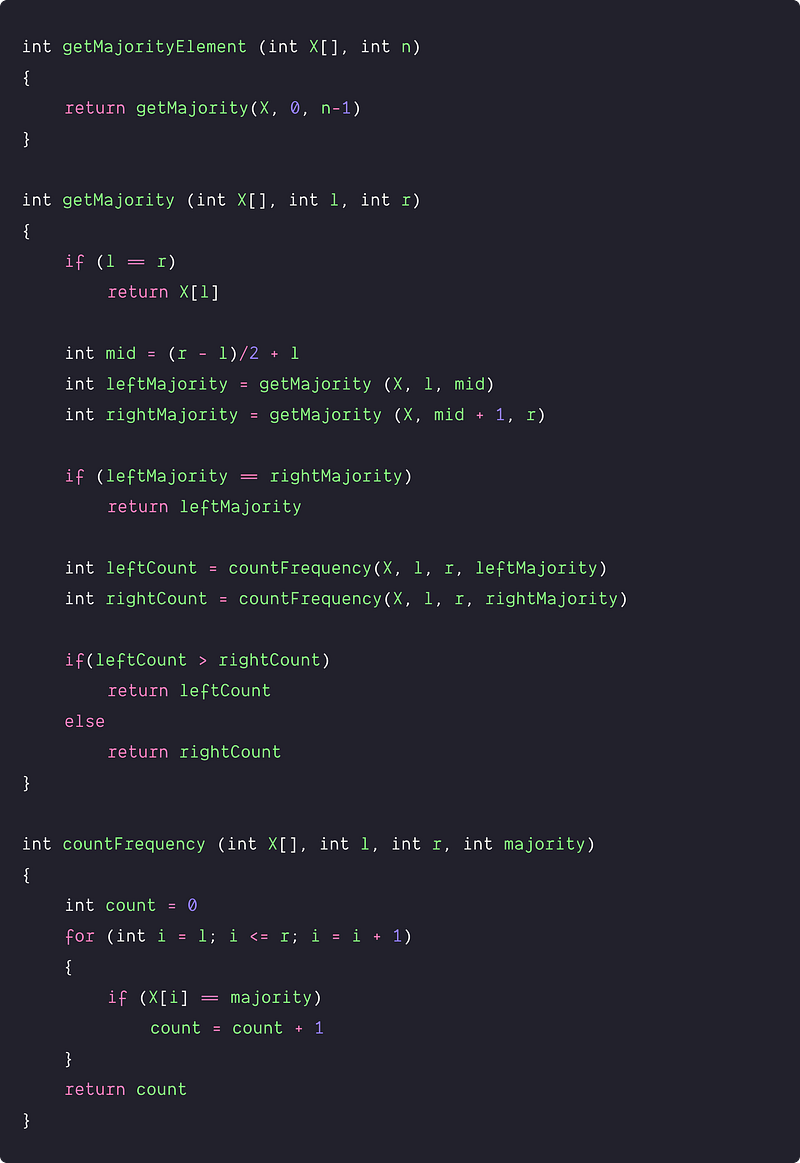Time and space complexity analysis

We are solving the problem of size n by recursively solving the two smaller sub-problem of size n/2 and combing the solution of these two smaller problems by counting the frequency of the leftMajority and rightMajority (by performing two linear scans of size n).

• The time complexity of the divide part = O(1)
• The time complexity of the conquer part = 2 T(n/2)
• The time complexity of the combine part = Counting the frequency of leftMajority + Counting the frequency of rightMajority = O(n) + O(n)
• Overall time complexity T(n) = O(1) + 2T(n/2) + O(n) = 2T(n/2) +O(n)

So the time complexity can be represented by the following recurrence relation :

``````T(n) = 2 T(n/2) + O(n), if n > 1

T(n) = O(1), if n = 1
``````

We can use the master theorem or recursion tree method to solve this recurrence relation. If we observe closely, this is similar to the merge sort recurrence. Time Complexity = O(nlogn) in the worst case.

Space complexity = O (logn) for recursion call stack. The divide & conquer method does not explicitly allocate any additional memory, but it uses an additional memory in stack frames due to recursion. The stack frame size depends on the max depth of the recursion tree, which is equal to O(logn) here. (Think!)

### Using a hash table

Solution Idea

In the brute force approach, we are counting the frequency of each element using the inner loop. Can we remove this inner loop and count the frequency in O(1)? Yes, the idea would be to use a hash table to count and store the frequency of each distinct element. Hash Table allows us to access and insert elements in O(1) on average.

Solution Steps

1. Create a hash table of size O(n) to store the frequency for each distinct element in the array. We store this as a key-value pair, i.e. (element, frequency) pair. Initially, all the slots in the hash table will be initialized with 0.
2. Now traverse the array from i = o to n - 1 to search X[i] in the hash table.
3. If X[i] is not present then we insert it into the hash table and update the frequency equal to 1. Otherwise, we increase its frequency by 1.
4. Now we check the frequency of the element X[i] stored in the hash table. If the frequency of X[i] is greater than n/2, then return x[i] as a majority element.

Solution Pseudocode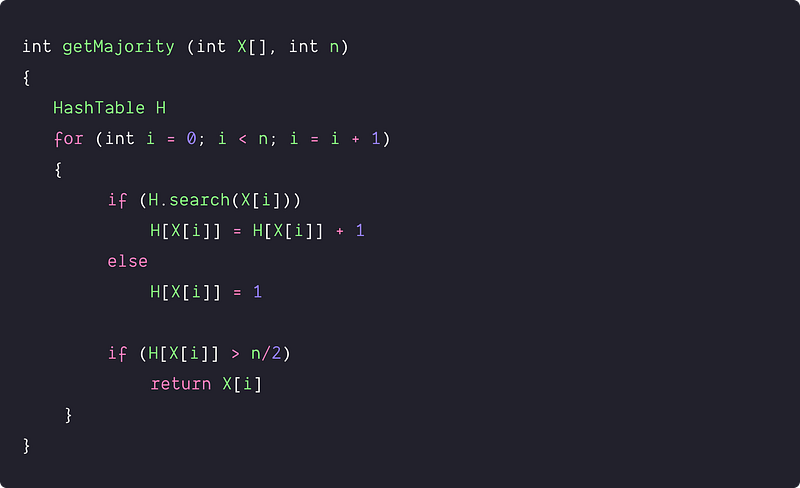Time and space complexity analysis

We are traversing the array once and performing constant time hash table operations on each iteration. Therefore, the time complexity = O(n). Space complexity = O(n) for the Hash Table.

### Using bit manipulation approach

Solution Idea

We can use mathematical insights from the binary representation of the numbers to find the majority element. How? Let's think.

Assume that each element in the array is a 32-bit integer. As given in the problem, we also know that there is a majority element in the array. Now for all numbers in the array, we count the frequency of set bits i.e. count the frequency of 1's at each bit position in the bitwise representation from 1 to 32. It's like polling for each bit position. At any position in the bitwise representation:

• If the frequency of 1's is in the majority (greater than n/2), then the bit value at the same position in the majority element will be 1.
• If the frequency of 1's is not in the majority (less than n/2), then the bit value at the same position in the majority element will be 0.

For example, X[ ] = [6, 13, 13, 2, 13], n = 5, let's assume our input is 4-bit integer.

``````6  ->      0 1 1 0

13 ->      1 1 0 1

13 ->      1 1 0 1

2  ->      0 0 1 0

13 ->      1 1 0 1
-----------------------
Majority = 1 1 0 1 = 13
``````

In the above example, let's start from left to right. 1's are in the majority at 1st, 2nd, and 4th position, so set bits at the similar position in majority element would be 1. At the 3rd position, the number of 1's across all array elements is only 2, not a majority, so it was set to 0 in the majority element.

So the idea would be simple - by identifying the majority bits at every bit position for all the numbers in the array, we can bitwise construct the majority element. But how do we implement it? Let's think.

Solution Steps

Initialize the value of the majority element, i.e., majorityElement = 0. Initially, all the bits in the majorityElement would be 0. We run a loop from currBit = 0 to 31, where the loop variable currBit represents the current bit position.

• For each bit position, initialize a variable to count the frequency of 1's i.e. count_ones = 0.
• Now iterate over the entire array element and increase count_ones by one if the current bit position is 1 in an element i.e.

``````for(i = 0; i < n; i = i + 1)
{
if((X[i] &(1<<currBit)) != 0)
count_ones = count_ones + 1
}
``````
• By end of the loop, if the count_ones value is greater than n/2, then we set the current bit position as 1 in the variable majorityElement. Otherwise, we leave it because bit at that position is already set 0 in the majorityElement.

``````if(count_ones > n/2)
majorityElement = majorityElement + (1 << currBit)
``````
• By the end of the loop, we return the value stored in majorityElement.

Solution Pseudocode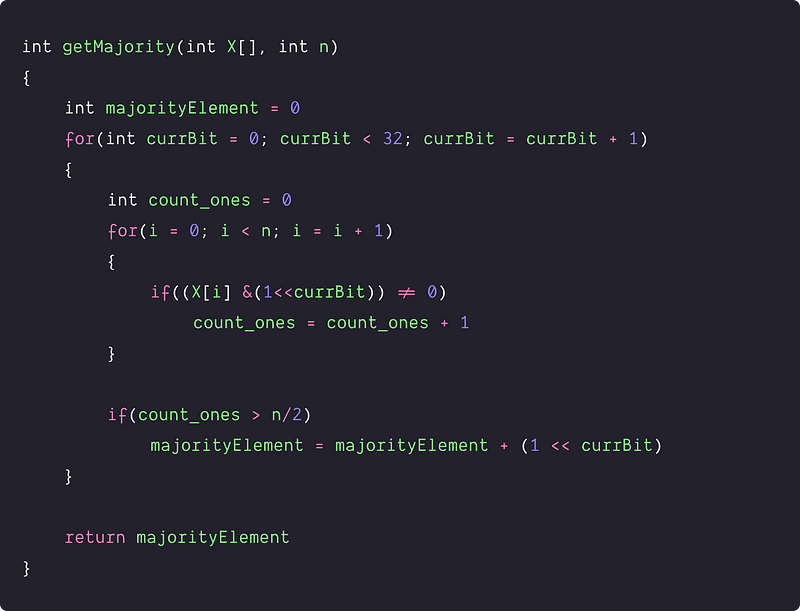Time and space complexity analysis

We are running two nested loops where the inner loop is running n times and the outer loop is running 32 times or constant time. Other than this, we are doing a constant number of operations. Time Complexity = 32 * O(n) = O(n),

Space Complexity = O(1), we are using a constant number of variables only.

### Using a randomized algorithm

Solution Idea

Here is the intuition of the randomized algorithm :  more than n/2 elements are equal to the majority element and a randomly picked element has more than 50% probability of success. So, we just select a random element in the array and check whether it is the majority element or not. return if it is, and repeat the same process if it is not.

But there are some critical questions : what is the exact probability that the algorithm will find the repeated elements? What is the running time of the algorithm? how a randomized algorithm is a good choice? (Think!)We can get an answer to this question in the analysis section.

Solution Pseudocode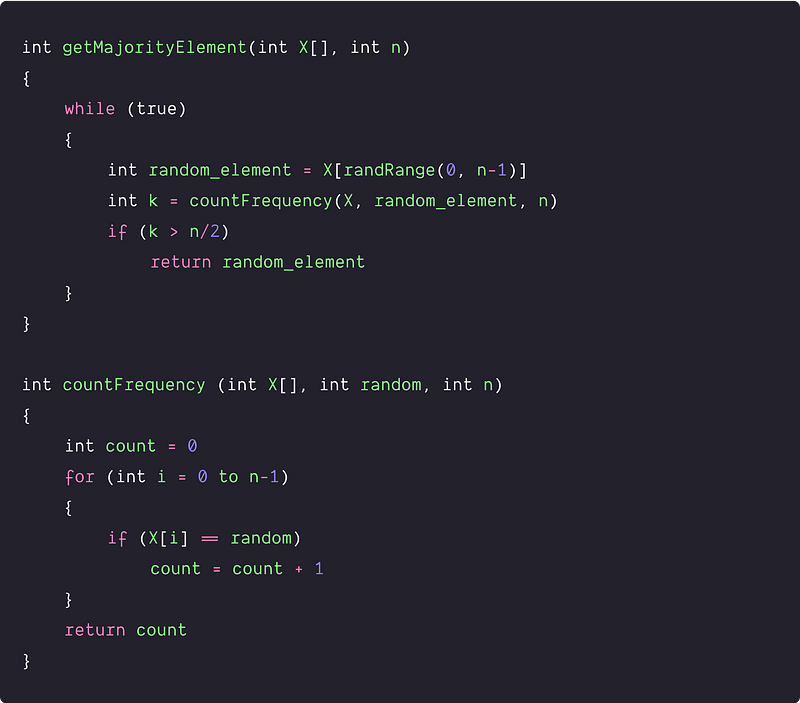Time and space complexity analysis

• There are n choices for picking an element in the array and out of these choices the chances of success are n/2+1 in the worst case. Suppose every choice is equally likely, then the probability of success is = (n/2 +1)/n = 1/2 + 1/n > 1/2 (for n>0). In we can say in other words, the probability of failure is at most 1/2.
• For reducing the probability of failure, we are repeating the algorithm again, if we don’t succeed. Each time we are making independent random choices. If we repeat the algorithm 10 times, then the probability of failure < (1/2)¹⁰
• If we repeat the algorithm logn times, then the probability of failure < (1/2)^logn = 1/(n^log 2) = 1/n. In other words, the algorithm succeeds with high probability i.e. at least (1–1/n). If the value of n is very large, then this algorithm can produce the majority element in O (logn) time complexity with a high probability.
• If we repeat the algorithm n times, then the probability of failure < (1/2)^n = 1/(2^n). In other words, the algorithm succeeds with high probability i.e. at least 1 – 1/(2^n) where c>0.

Note: The idea of “with high probability” is often used in the analysis of the randomized algorithms, where the “high probability” is with respect to the input size n.

### An efficient approach: The Boyer-Moore voting algorithm

Solution Idea

Here is the intuition behind this algorithm — Since the majority element occurs more than n/2 times, its frequency is greater than all other elements combined. Therefore, if we mark the occurrence of the majority element as +1 and the occurrence of any other element as -1, then the overall sum of them would be definitely greater than zero (Think!)

Here is an interesting analogy to understand the algorithm: suppose we have n number of people, each holding one element of the array. Then, whenever two people find each other where neither holds the same array element as the other, they sit down. Eventually, in the end, if anyone is left standing, then that element is the majority element. Since the majority element occurs more than n/2 times, you can guarantee that this approach will always find the majority element.

Solution Steps

• To keep track of our current guess of the majority element, we declare the variable majorityCandidate and maintain a variable counter. Initially, the value of both variables is equal to 0.
• Let’s walk across the array, if the current element matches our guess, we increment the counter by1. If the current element doesn’t match our guess, then we decrement the counter by 1. (Think!)
• If the value of the counter is equal to 0, then we reset the current guess and make it equal to the current element. i.e majorityCandidate = X[i]. In other words, we forget about everything up to the previous index and consider the current element as the potential candidate for the majority element in the array.
• By the end of the loop, the value of the majority element gets stored in the variable majorityCandidate.

There are many ways to think about why this algorithm works. One good intuition is to think of the algorithm as breaking the input down into a chunk of consecutive copies of particular values. Incrementing the counter then corresponds to marking that multiple copies of the same value while decrementing it corresponds to some other sequences of values, “canceling out” the accumulation of values of a particular type.

Note: As long as the majority element occurs with a frequency of at least n/2, we can guarantee that this approach will always find the majority element.

Solution Pseudocode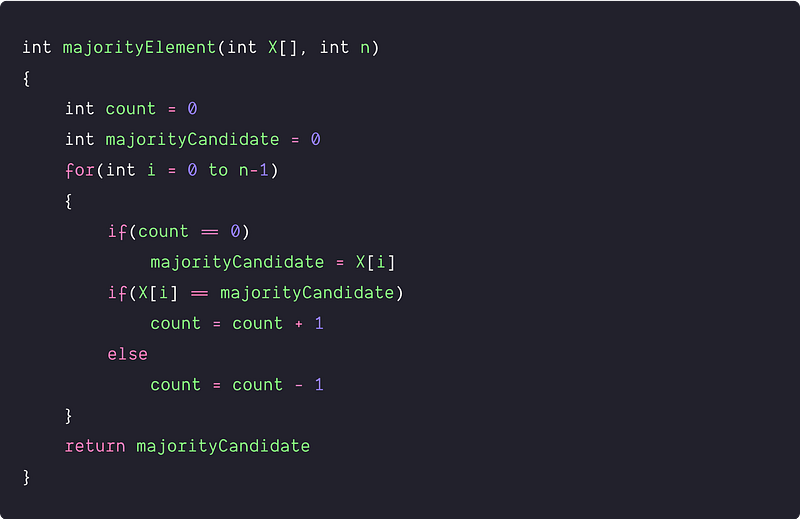Time and space complexity analysis

We are running a single loop at n time and performing the constant operation at each step of the iteration. Time Complexity = O(n). Space complexity = O(1) because we are using constant extra space.

### Critical ideas to think!

• How do we modify the solution approaches if the majority element does not always exist in the array?
• Can this problem be solved using a Binary Search Tree? What will be the time and space complexity?
• How do we implement the randRange( ) function in the 6th approach?
• Prove the correctness of the Boyer-Moore Majority Vote Algorithm.
• What is the best and worst-case space complexity in the 4th approach?

### Comparisons of time and space complexities

• Two nested loops: Time = O(n^2), Space = O(1)
• Using Sorting: Time = O(nlogn), Space = O(1)
• Divide and Conquer: Time = O(nlogn), Space = O(1)
• Hash Table: Time = O(n), Space = O(n)
• Bit manipulation: Time = O(n), Space= O(1)
• Randomized algorithm: Time = O(logn), with a probability of failure 1/n if n is very large, Space = O(1)
• Boyer-Moore Voting algorithm: Time = O(n), Space = O(1)

### Suggested coding questions to practice

• Find if any element repeats more than n/3 times
• Check if an array has a majority element
• n Repeated element in 2n size array
• Find the majority element in a circular array of 0’s and 1’s
• Sort elements based on their frequency
• Find the element with the second-largest frequency

Enjoy learning, Enjoy coding, Enjoy algorithms!

Get well-designed application and interview centirc content on ds-algorithms, machine learning, system design and oops. Content will be delivered weekly.